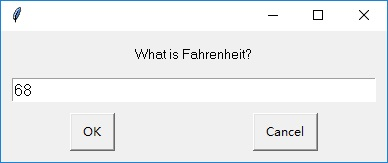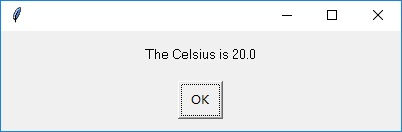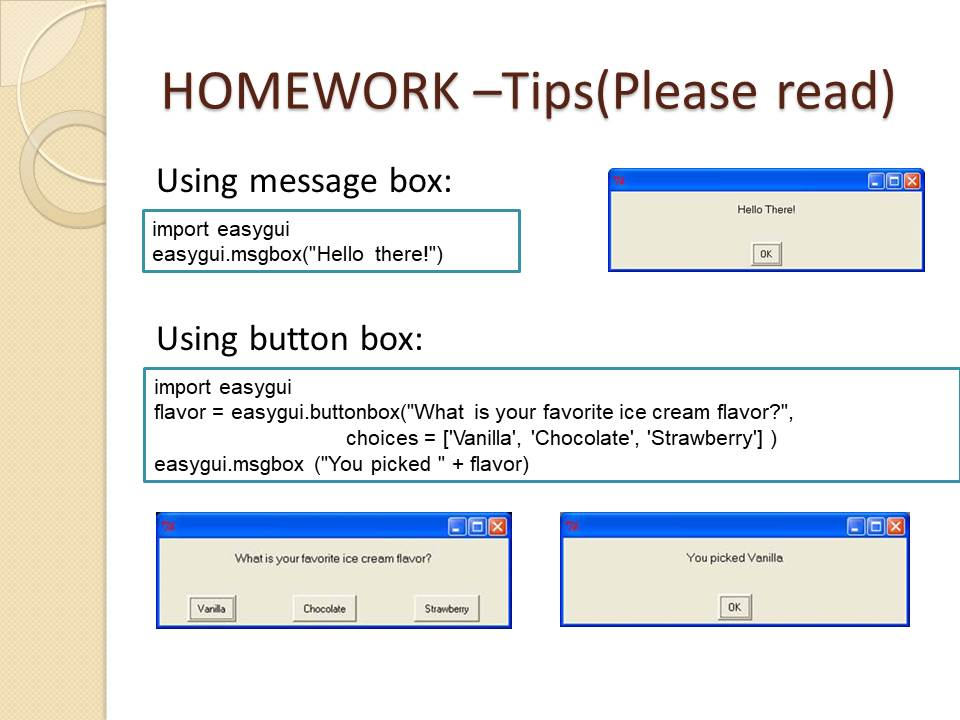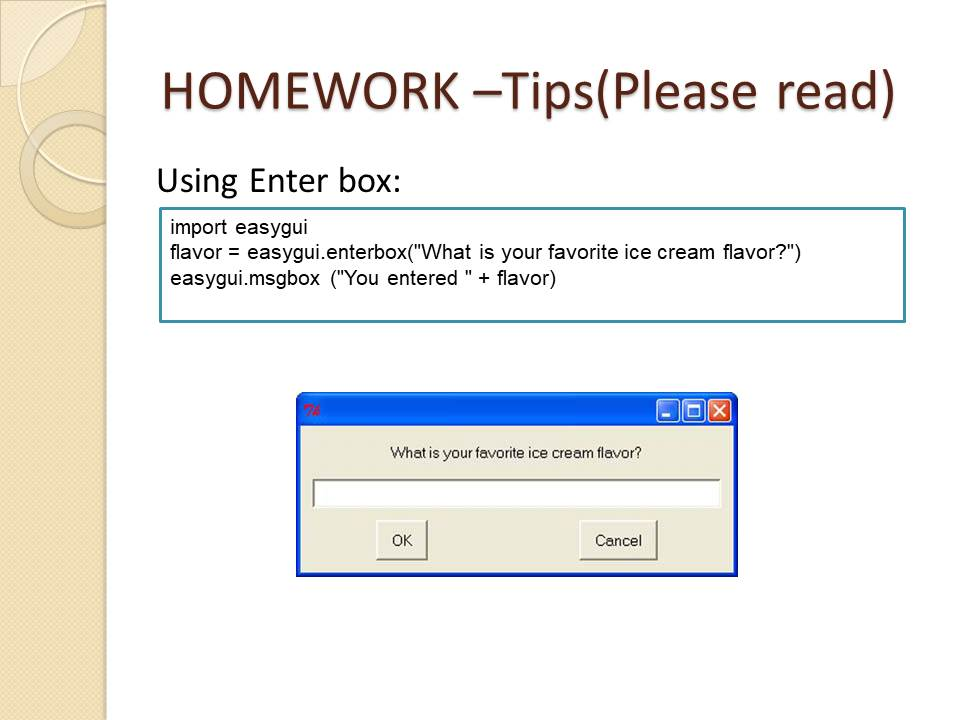TORONTO KIDS COMPUTER CLUB | TCMS 18:30 Python Homework 11.08
16708

# TCMS 18:30 Python Homework 11.08

## 13 Nov TCMS 18:30 Python Homework 11.08

Question 1

Use EasyGui to write a program to convert temperatures from Fahrenheit to Celsius. The formula for that is: Celsius = 5 / 9 * (Fahrenheit – 32). Use GUI input and output. You need to create a easygui enterbox to ask Fahrenheit, then use message box to show the Celsius degree. (Hint: the input you get from the enter box is a string, so you need float() to convert it into decimal number.)Question 2:
Use EasyGui to create a program which asks the user to enter two numbers and display the sum, using two enterboxes and a msgbox.

Class review and Hint: